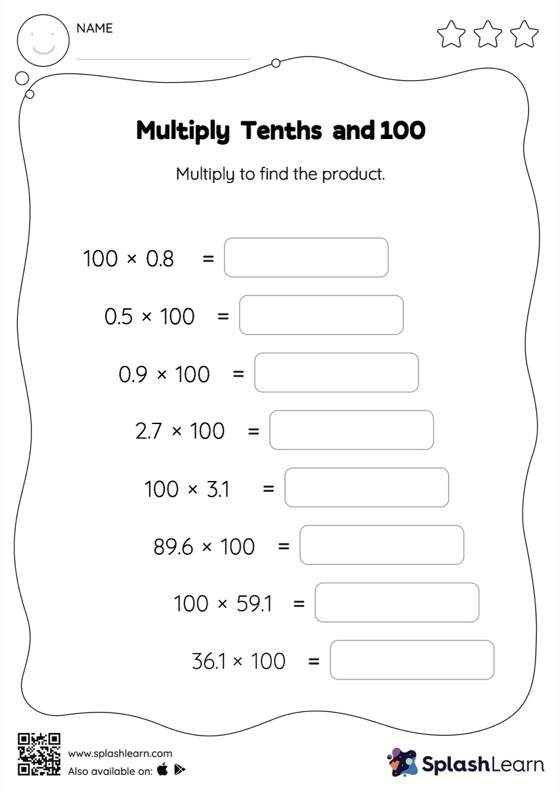# Multiply Tenths and 100: Horizontal Multiplication Worksheet

Home > Multiply Tenths and 100: Horizontal MultiplicationDoes your child know how to multiply tenths and 100? Invite them to practice this concept here. When a decimal is multiplied by 100, the decimal point moves two places to the right. Students use this understanding to multiply tenths and 100 in this worksheet. In this worksheet, students practice solving problems written in the horizontal format. How numbers are laid out in a problem affects the method a student employs to solve it. Therefore to develop actual fluency and mastery of multiple strategies, students must practice different formats.